Open in App
Not now

# Introduction to Arrays – Data Structure and Algorithm Tutorials

• Last Updated : 21 Dec, 2022

## What is an Array?

An array is a collection of items of the same variable type stored that are stored at contiguous memory locations. It’s one of the most popular and simple data structures and is often used to implement other data structures. Each item in an array is indexed starting with 0.

The dream of every programmer is to become not just a good, but also a great programmer. We all want to achieve our goals and to achieve our goals, we must have a great plan with us. In this context, we have decided to provide a complete guide for Arrays interview preparation, which will help you to tackle the problems that are mostly asked in the interview, such as What is an Array, What is Array in C language, How do you initialize an Array in C, How to sort an Array, etc. We have also covered the topics such as Top Theoretical interview questions and Top interview coding questions in this complete guide for Array interview preparation.Complete guide for Arrays interview preparation

We can directly access an array element by using its index value.

## Basic terminologies of array

• Array Index: In an array, elements are identified by their indexes. Array index starts from 0.
• Array element: Elements are items stored in an array and can be accessed by their index.
• Array Length: The length of an array is determined by the number of elements it can contain.

## Representation of Array

The representation of an array can be defined by its declaration. A declaration means allocating memory for an array of a given size.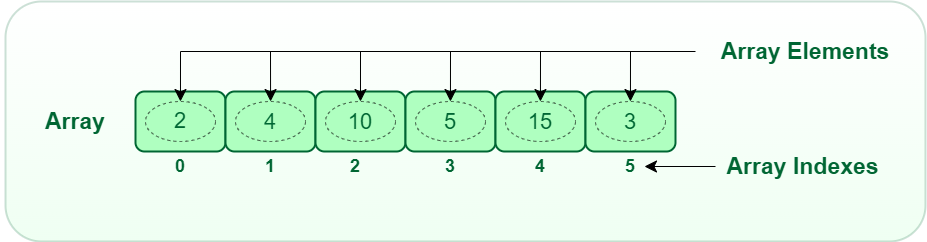Array

Arrays can be declared in various ways in different languages. For better illustration, below are some language-specific array declarations.

## C++

 `int` `arr;        ``// This array will store integer type element` `char` `arr;    ``// This array will store char type element` `float` `arr;  ``// This array will store float type element`

## C

 `int` `arr;        ``// This array will store integer type element` `char` `arr;    ``// This array will store char type element` `float` `arr;  ``// This array will store float type element`

## Java

 `/* Static Arrays are arrays that are declared before runtime` `and are assigned values while writing the code.` `*/`   `// The syntax of declaring a static array is:` `[]` `    ``= {, ,….. };`   `// Example:` `int` `arr[] = { ``2``, ``5``, ``6``, ``9``, ``7``, ``4``, ``3` `};`

## C#

 `int``[] arr = ``new` `int``; ``// This array will store integer type element`

## Javascript

 `// JS code` `let arr=[10,20,30];  ``// This array will store integer` `let arr2 = [``'c'``, ``'d'``, ``'e'``] ``// This array will store characters` `let arr3 = [28.5, 36.5, 40.2] ``// This array will store floating elements`

## Python

 `# Python code` `arr ``=` `[``10``, ``20``, ``30``]  ``# This array will store integer` `arr2 ``=` `[``'c'``, ``'d'``, ``'e'``]  ``# This array will store characters` `arr3 ``=` `[``28.5``, ``36.5``, ``40.2``]  ``# This array will store floating elements`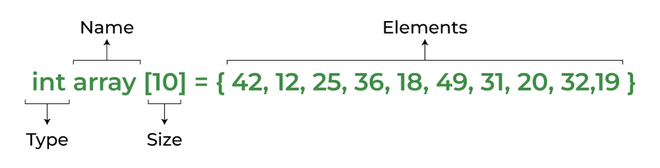Array declaration

However, the above declaration is static or compile-time memory allocation, which means that the array element’s memory is allocated when a program is compiled. Here only a fixed size (i,e. the size that is mentioned in square brackets []) of memory will be allocated for storage, but don’t you think it will not be the same situation as we know the size of the array every time, there might be a case where we don’t know the size of the array. If we declare a larger size and store a lesser number of elements will result in a wastage of memory or either be a case where we declare a lesser size then we won’t get enough memory to store the rest of the elements. In such cases, static memory allocation is not preferred.

### Is it possible to create dynamic array?

The answer is Yes. It is possible to allocate memory dynamically. So, dynamic memory allocation is the process of assigning the memory space during the execution time or the run time.

Below are the languages that support dynamic memory allocation:

## C++

 `int` `*array = ``new` `int``;`

## Java

 `// The syntax of declaring a dynamic array is:`   `// [ ];` `// Example: ` `int` `arr[``10``]; ``// Store integer elements` `String arr[``5``]; ``// Store String type of elements`

## Python3

 `# list of integers` `my_list ``=` `[``1``, ``2``, ``3``, ``4``]`   `# Empty list` `my_list ``=` `[]`   `# list of mixed data types` `my_list ``=` `[``"Hello"``, ``1``, ``5.5``]`

## C#

 `int``[] numArray = ``new` `int``[] {};`

## Javascript

 `// JavaScript has dynamic arrays: their size is not predetermined, nor the type of data.`   ` ``// Create array using literal` `var` `x = [``"a"``, ``"b"``, ``"c"``];   `   `// Create array using constructor` `var` `x = ``new` `Array();        `   `// Create array using constructor with items` `var` `y = ``new` `Array(``"a"``, ``"b"``, ``"c"``);`

## PHP

 `\$arr` `= ``array``(``"a"``,``"b"``,``"c"``);`

## Why Array Data Structures is needed?

Assume there is a class of five students and if we have to keep records of their marks in examination then, we can do this by declaring five variables individual and keeping track of records but what if the number of students becomes very large, it would be challenging to manipulate and maintain the data.

What it means is that, we can use normal variables (v1, v2, v3, ..) when we have a small number of objects. But if we want to store a large number of instances, it becomes difficult to manage them with normal variables. The idea of an array is to represent many instances in one variable..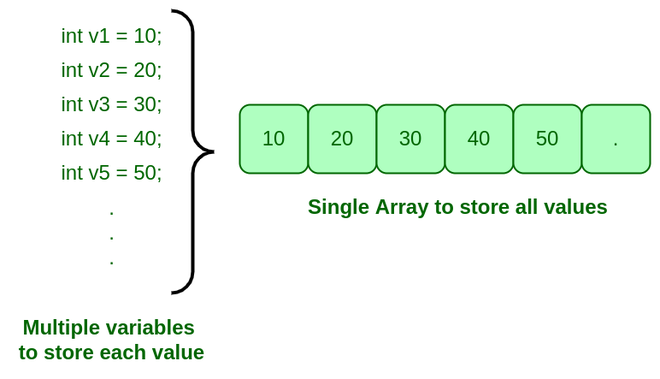Need for Array

## Types of arrays:

There are majorly two types of arrays: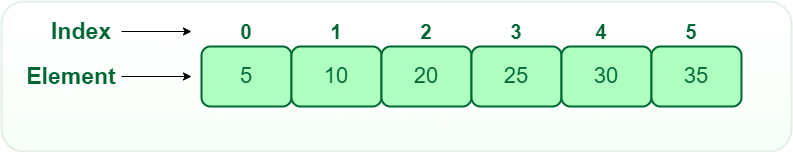1D array

• Two-dimensional array: 2-D Multidimensional arrays can be considered as an array of arrays or as a matrix consisting of rows and columns.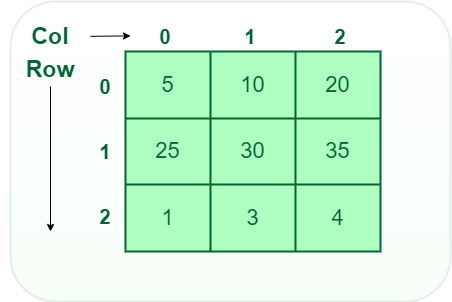2D array

• Three-dimensional array: A 3-D Multidimensional array contains three dimensions, so it can be considered an array of two-dimensional arrays.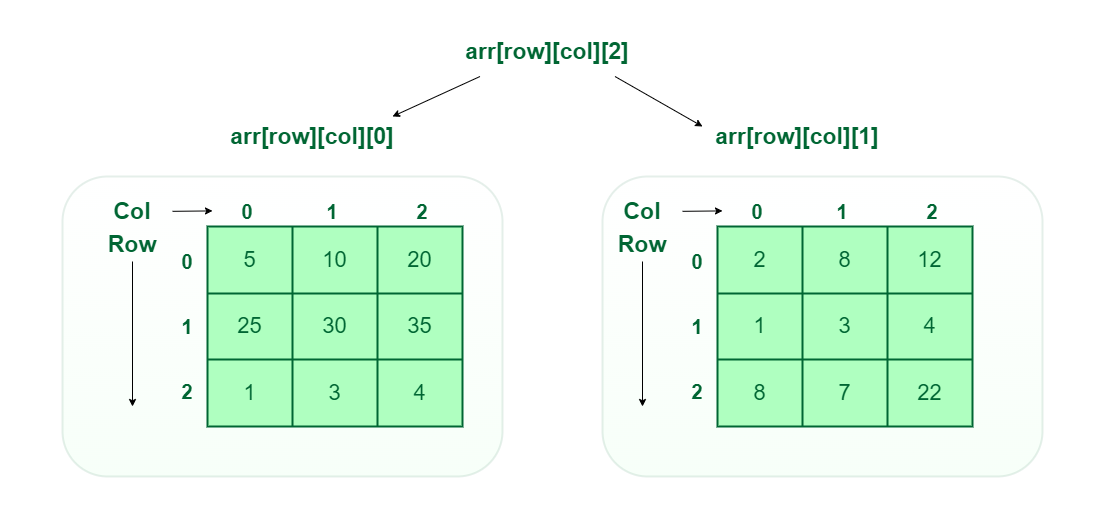3D array

## Types of Array operations:

• Traversal: Traverse through the elements of an array.
• Insertion: Inserting a new element in an array.
• Deletion: Deleting element from the array.
• Searching:  Search for an element in the array.
• Sorting: Maintaining the order of elements in the array.

• Arrays allow random access to elements. This makes accessing elements by position faster.
• Arrays have better cache locality which makes a pretty big difference in performance.
• Arrays represent multiple data items of the same type using a single name.
• Arrays store multiple data of similar types with the same name.
• Array data structures are used to implement the other data structures like linked lists, stacks, queues, trees, graphs, etc.

• As arrays have a fixed size, once the memory is allocated to them, it cannot be increased or decreased, making it impossible to store extra data if required. An array of fixed size is referred to as a static array.
• Allocating less memory than required to an array leads to loss of data.
An array is homogeneous in nature so, a single array cannot store values of different data types.
• Arrays store data in contiguous memory locations, which makes deletion and insertion very difficult to implement. This problem is overcome by implementing linked lists, which allow elements to be accessed sequentially.

## Application of Array:

• They are used in the implementation of other data structures such as array lists, heaps, hash tables, vectors, and matrices.
• Database records are usually implemented as arrays.
• It is used in lookup tables by computer.
• It is used for different sorting algorithms such as bubble sort insertion sort, merge sort, and quick sort.

## Top 50 interview coding question

### 1. What is an array in data structure with example?

An array is a collection of items of the same data type stored at contiguous memory locations. Ex. int arr = {1,2,3,4,5};

### 2. Why array is a data structure?

Arrays store elements of the same type, they are classified as homogeneous data structures. They can store numbers, strings, characters, boolean values (true and false), objects, and so on.

### 3. What data structure is an array?

An array is a linear data structure that stores similar elements in contiguous memory locations.

### 4. What are the types of arrays?

There are majorly two types of arrays:

• One dimensional array
• Multidimensional array

### 5. How is data stored in an array?

An array is a collection of items of the same data type stored at contiguous memory locations or says the elements are stored one after another in memory. An array uses an index system starting at 0 and going to (n-1), where n is its size.

### 6. Difference between array and structure?

The structure can contain variables of different types but an array only contains variables of the same type.

### 7. What are the limitations of an array?

An array is a collection of items of the same data type, That means, in an integer array only integer values can be stored, while in a float array only floating values and character array can have only characters. Thus, no array can have values of two data types.

### 8. What are the advantages of an array?

There are multiple advantages of array data structure and some of them are:

• Arrays allow random access to elements. This makes accessing elements by position faster.
• Arrays store multiple data of similar types with the same name.
• Array data structures are used to implement the other data structures like linked lists, stacks, queues, trees, graphs, etc.

### 9. What is the purpose of using arrays?

An array is used when several variables of the same type need to be used, and it can be defined as a sequence of objects of the same type.

### 10. What is a multidimensional array?

A multi-dimensional array can be termed as an array of arrays that stores homogeneous data in tabular form. Data in Multidimensional Arrays are stored in row-major order.

## Conclusion

After the discussion, we concluded that arrays are a simple method of accessing elements of the same type by grouping them and we can find the elements efficiently by their indexes and can perform different operations using them. Thus, they are more efficient when it comes to memory allocation and should be used in all modern programming languages. So, this becomes a favorite topic for the perspective of the interview and most of the companies generally asked about the problems on the array. For all these reasons, we must have a good knowledge of it.

Related articles:

My Personal Notes arrow_drop_up
Related Articles